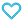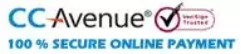# NCERT Textbook + Exemplar Problems Solutions Mathematics Class 12

400.00

• Paper Book

MTG’s NCERT Textbook + Exemplar Problem solutions- Mathematics comprises of detailed and error free solutions of all the questions of NCERT Textbook and NCERT Exemplar Book. By practicing these questions students will develop analytical approach to solve problems.

858 in stock

Qty:Add to WishlistMTG’s NCERT Textbook + Exemplar Problem solutions- Mathematics is an excellent book designed to provide a comprehensive guide to NCERT Textbook and NCERT Exemplar Problems of Mathematics for Class XII students. This book provides a detailed explanation to all subjective and objective type questions given in both NCERT Textbook and NCERT Exemplar Problems book. This is an error free and ‘Self-study’ book to master the concepts in Mathematics.

This book aims to give the best solutions and help the students to score high in school examinations as well as to build a strong foundation for Olympiads and competitive exams like PETs & PMTs.

Table of Content:-
• 1. Relations and Functions
• 2. Inverse Trigonometric Functions
• 3. Matrices
• 4. Determinants
• 5. Continuity and Differentiability
• 6. Application of Derivatives
• 7. Integrals
• 8. Application of Integrals
• 9. Differential Equations
• 10. Vector Algebra
• 11. Three Dimensional Geometry
• 12. Linear Programming
• 13. Probability
ISBN11 9789389167481 MTG Editorial Board 2019-20 548 Class 12 School Books Mathematics 682gm

## Reviews

There are no reviews yet.